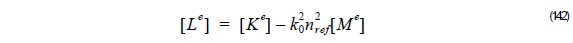Using the recurrence formula Equation 118, we get:Here a = 2k0nref .

Using Equation 119 into Equation 131, we get:Thus,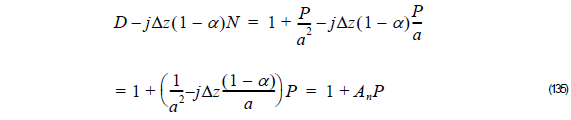and,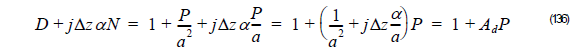whereThus, we can get the unknown field φ l + 1  at z + Δz from the known field φ l  solving the triagonal linear system:If we consider FEM we get,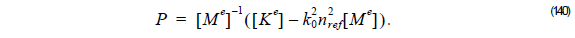Thus, we can rewrite Equation 140 as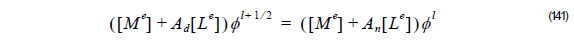here